# Why #DIV/0! occur And How to Fix #DIV/0! in Excel

In this article, we will learn about why #DIV/0! occur And How to Fix #DIV/0! in Excel.

In excel, many times we get #DIV/0! error. This error occurs when there is a mathematical error in the formula you have supplied to the cell and is recognized by Excel by showing the error. As per Microsoft official site a #DIV/0! is Excel's way of saying, There's something wrong with  input formula values which indeed resulting the error.

Mathematical error like division of a number with Zero.

In Excel any number when divided by zero returns #DIV/0! Error.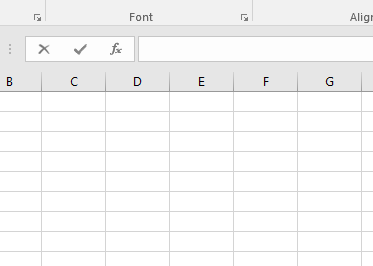As you can see from the above gif that dividing any number with zero returns #DIV/0! Error.

Let’s understand how this error occur and how to fix them with some examples

## #DIV/0! error due to blank cell.

The most basic reason of #DIV/0! error is when blank cell is provided as the argument to the function. Generally Excel understand Blank cells as value equal to zero except with some functions.

For example,  QUOTIENT function takes the two arguments both numbers. But here the function returns error as the cell is blank.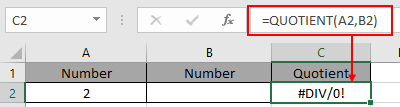Look in the above snapshot and realize the mathematical mistake just happened. The above function divides 2 by zero.

How to fix it! You cannot fix the error for the result. But you can catch errors and extract results on the basis of it.

two ways to catch the blank cells which returns the #DIV/0! Error

First method using IF and ISBLANK function.

Use the formula:

 = IF ( ISBLANK ( cell_reference ) , "The denominator is blank" , QUOTIENT ( number1 , number2 ) )

Explanation:

• IF function first checks the condition and returns value_if_true or else value_if_False.
• ISBLANK function catches the blank cell and returns the string "The denominator is blank" if TRUE.
• ISBLANK function checks for blank cell in cell reference and returns the formula QUOTIENT ( number1 , number2 ) ) if FALSE.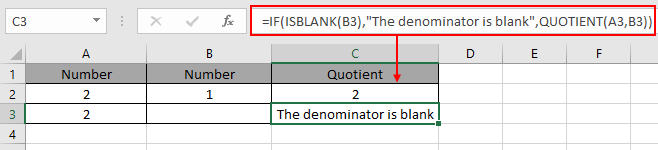There's same formula in C2 and C3 cell.

Second method is using the IFERROR function.

Explanation:

• IFERROR function checks for any error where the operation is performed in this cas  QUOTIENT ( A3 , B3 ).
• If the operation performed returns error the function returns the string "The denominator is blank".
• If the operation performed returns a value the function returns the value.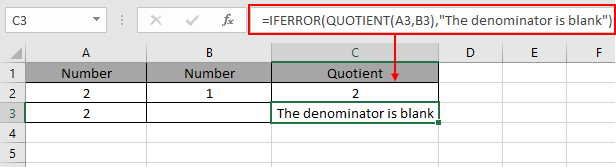As you can see the #DIV/0! Error when operating with blank cells.

## #DIV/0! error due to text (considered as zero) in cells

Sometimes, the functions which takes numerical value are fed with text value, then this #DIV/0! Error occur.

For example,  AVERAGE function in excel takes numbers as arguments and returns their AVERAGE.

AVERAGE = Sum of values / total number of Values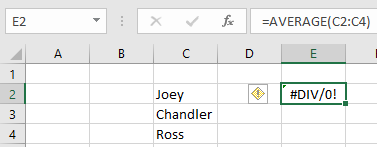Or when the numbers as given in text format.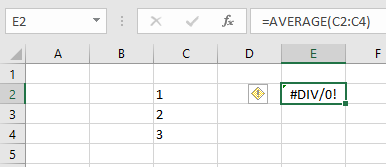Look in the above snapshot and realize the mathematical mistake just happened. The above function gets text instead of numbers. So the function returns #DIV/0! error.

How to fix it! You cannot fix the error for the result unless you give numbers instead of text.

two ways to catch the blank cells which returns the #DIV/0! Error.

First method checking the argument that its TEXT or number. Use the Value function to first convert the text to number and then operate the function.

 = VALUE (cell_reference)

Explanation:
The VALUE function  converts the Value to its data type form. So that Excel can read the value with its correct format.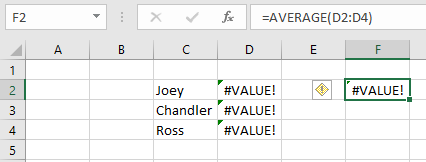Here The function generates #VALUE! Error, Excel way of saying check your argument's data type before feeding to the function. Learn more about #VALUE! Error here.

When this method is used with Numbers as text.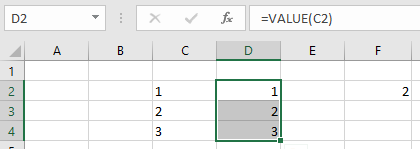First texts are converted to numbers. And then the average is taken on the numbers array

## Trapping multiple blank cells using IF & OR function

For example: Mathematical error like division of a number with Zero.

In Excel any number when divided by zero returns #DIV/0! Error.As you can see from the above gif that dividing any number with zero returns #DIV/0! Error.

Use the formula

 = IF ( OR ( A2 = "" , B2 = "" ), "" , A2/B2 )

Explanation:

OR function checks all the cell_reference, if all the condition stands TRUE, then function returns value_if_true or else returns value_if_false.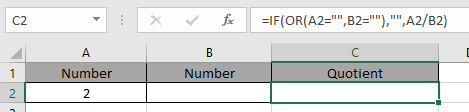As you can see how mathematical error with predefined array argument name returns #DIV/0! error and after the correction the formula works fine.

Hope this article about how Why #DIV/0! occur And How to Fix #DIV/0! error in Excel is explanatory. Find more articles on Excel errors functions here. Please share your query below in the comment box. We will assist you.

Related Articles

#VALUE Error And How to Fix It in Excel

How to correct a #NUM! Error

How to use the wildcards in excel

How to Remove Text in Excel Starting From a Position

Validation of text entries

Create drop down list in excel with colour

Remove leading and trailing spaces from text in Excel

Popular Articles

Edit a dropdown list

Absolute reference in Excel

If with conditional formatting

If with wildcards

Vlookup by date

Join first and last name in excel

Count cells which match either A or B

Convert Inches To Feet and Inches in Excel 2016

50 Excel Shortcut to Increase Your Productivity

Terms and Conditions of use

The applications/code on this site are distributed as is and without warranties or liability. In no event shall the owner of the copyrights, or the authors of the applications/code be liable for any loss of profit, any problems or any damage resulting from the use or evaluation of the applications/code.